# Precalculus : Evaluating Trig Functions

## Example Questions

2 Next →

### Example Question #6 : Find The Degree Measure Of An Angle For Which The Value Of A Trigonometric Function Is Known

Which of the following could be a value of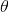?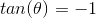Possible Answers: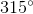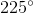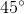Correct answer:Explanation:

Which of the following could be a value of?To begin, it will be helpful to recall the following property of tangent: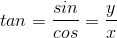This means that ifour sine and cosine must have equal absolute values, but with opposite signs.

The only place where we will have equal values for sine and cosine will be at the locations halfway between our quadrantal angles (axes). In other words, our answer will align with one of the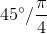angles.

Additionally, because our sine and cosine must have opposite signs (one negative and one positive), we need to be in either quadrant 2 or quadrant 4. There is only answer from either of those two, so our answer must be.

### Example Question #1 : Find The Degree Measure Of An Angle For Which The Value Of A Trigonometric Function Is Known

Findif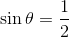and it is located in Quadrant I.

Possible Answers: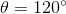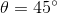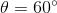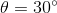Correct answer:Explanation:

Since we know the value of the trigonometric function and the triangle is located in Quadrant I, we can draw the triangle and get a sense of it. If the opposite side is 1 and the hypotenuse is 2, we know that we're dealing with a 30-60-90 special triangle. And since the opposite side of the angle is 1, we know that the angle is.

### Example Question #8 : Find The Degree Measure Of An Angle For Which The Value Of A Trigonometric Function Is Known

Given the equation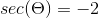, what is one possible value of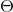?

Possible Answers: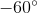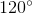Correct answer:Explanation:

Find 1 possible value ofGiven the following:Recall that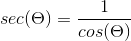So if, then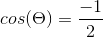Thinking back to our unit circle, recall that cosine corresponds to the x-value. Therefore, we must be in quadrants II or III.

So, which angles correspond to an x-value of -0.5? Well, they must be the angles closest to the y-axis, which are ourincrement angles.

This means our angle must be either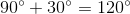or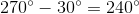It must be, because 240 is not an option.

Note that there are technically infinte solutions, because we are not given a specific interval. However, we only need to worry about one.

2 Next →

### All Precalculus Resources